## Digital Logic and Truth Tables Digital Logic and Truth Tables

### Information Processing Operations

How is digital information processed and manipulated?

We start with some fundamental operations on binary digits and work up to more complicated operations.

### AND

If x and y are binary digits (either 0 or 1) then

 x AND y
is a binary digit, defined by

 x AND y
 =
 1
 if x = 1 and y = 1
 =
 0
 otherwise
More explicitly, AND is defined by the table

 x
 y
 x AND y
 0
 0
 0
 0
 1
 0
 1
 0
 0
 1
 1
 1
Diagrammatically: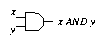The electronic component which implements the AND operation is called an AND gate.

### Truth Tables

Instead of 0 and 1, the binary values are sometimes referred to as false and true. The AND operation then has the same meaning as in ordinary language: x AND y is true if x is true and y is true.

The table defining AND is usually called a truth table, and can be written out using false and true instead of 0 and 1.

 x
 y
 x AND y
 false
 false
 false
 false
 true
 false
 true
 false
 false
 true
 true
 true
Yet another terminology is high and low for 1 and 0, referring to high and low voltage levels in electronics. We will use all these terms interchangeably, but we will normally stick to 0 and 1 when writing out truth tables.

### OR

If x and y are binary digits (either 0 or 1) then

 x OR y
is a binary digit, defined by

 x OR y
 =
 1
 if x = 1 or y = 1 or both
 =
 0
 otherwise
The truth table for OR is as follows.

 x
 y
 x OR y
 0
 0
 0
 0
 1
 1
 1
 0
 1
 1
 1
 1
Again, note that OR has the same meaning as in ordinary language: x OR y is true (i.e. 1) if x is true or y is true (or both).

Diagrammatically:### Example: Majority Voting

Imagine that three people have to vote either Yes (represented by 1) or No (represented by 0). The overall result is the majority decision.

If x, y, z stand for the three votes, and r stands for the result, then we can write

 r = (x AND y) OR (y AND z) OR (z AND x)
Diagrammatically:### NOT

It turns out that AND and OR are not sufficient to define all functions on binary digits. The missing ingredient is the NOT operation.

 x
 NOT x
 0
 1
 1
 0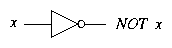Again, if we think in terms of truth values, NOT has its familiar meaning.

A NOT gate is often called an inverter.

By using AND , OR and NOT in combination, it is possible to define any desired function on binary numbers - for example, addition or multiplication. We will see how to do this in a few lectures' time.

Perhaps surprisingly, we only need NOT and just one of AND and OR . We can check that the circuit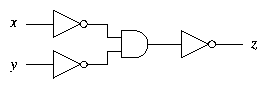calculates z = x OR y.

### One Fundamental Operation

Even more remarkably, it is possible to build up all functions from combinations of just one operation: the NAND operation, defined by

 x
 y
 x NAND y
 0
 0
 1
 0
 1
 1
 1
 0
 1
 1
 1
 0
and drawn like this: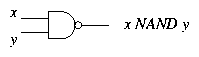You can check that

 x NAND y = NOT (x AND y).
Assuming that we have NAND but not AND , OR or NOT , we can define the other operations as follows:

 NOT x
 =
 x NAND x
 x AND y
 =
 NOT (x NAND y)
 x OR y
 =
 (NOT x) NAND (NOT y)

### XOR

In English, it is not always clear whether ``A or B'' means ``A or B or possibly both'' or ``either A or B but not both''. As we have seen, the logical operation OR has the first meaning; it is sometimes called inclusive or.

The operation with the second meaning is exclusive or, written XOR . If x and y are binary digits (either 0 or 1) then

 x XOR y
is a binary digit, defined by

 x XOR y
 =
 1
 if either x = 1 or y = 1 but not both
 =
 0
 otherwise
The truth table for XOR is as follows.

 x
 y
 x XOR y
 0
 0
 0
 0
 1
 1
 1
 0
 1
 1
 1
 0
Diagrammatically: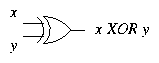### Another Fundamental Operation

Just as NAND is defined by

 x NAND y = NOT (x AND y),
we can also define an operation called NOR by

 x NOR y = NOT (x OR y).
Its truth table is as follows.

 x
 y
 x NOR y
 0
 0
 1
 0
 1
 0
 1
 0
 0
 1
 1
 0
It is drawn like this: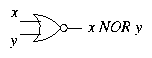Like NAND , NOR can be used to define all the other operations.

 NOT x
 =
 x NOR x
 x OR y
 =
 NOT (x NOR y)
 x AND y
 =
 (NOT x) NOR (NOT y)
Exercise: Check these equations.

### Truth Table Exercise

For practice in calculating the output from a circuit, use the TruthTable exercise.
This exercise can be found at the following page : TruthTable Exercise

File translated from TEX by TTH, version 2.78.
On 27 Jul 2001, 10:19.